#### Selecting the Proper Injector Size

• In order to select the correct size injector for your application, you might wish to use one of the following formulas.

• In most cases a naturally aspirated engine will have a B.S.F.C of .50. This means that the engine will use .50 lbs. of fuel per hour for each horsepower it produces. Turbocharged engines will want to be at .60 lbs. per hour or higher.

• Using these numbers as a guideline, you can select the approximate injector size in the following formula for a four cylinder, naturally aspirated engine at 200 bhp.

• Most injectors will max out at 80% duty cycle and this is the accepted industry standard.

• B.S.F.C is brake specific fuel consumption - How much fuel you are using per horsepower per hour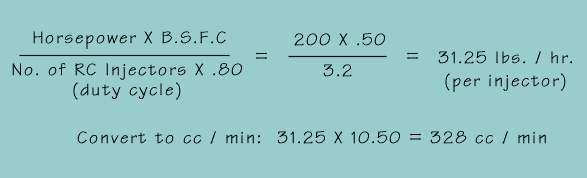The proper injector for this application is 31 lbs. / hr. or 330 cc / min
(Use the Fuel Injector Worksheet below to perform this calculation for you.)

To find the HP value of the of the above mentioned injectors use this formula: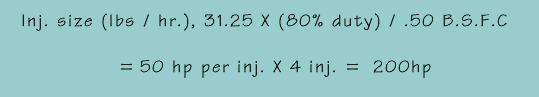#### Find Fuel Flow After Pressure Change

###### Calculate flow rate on pressure change

(cc/min or lb/hr)

The formula to find the new FUEL FLOW rate after a fuel pressure change: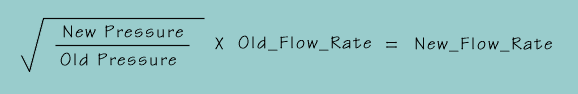Example 1:
A 240 CC injector tested at 43.5 PSI is run at 50 PSI.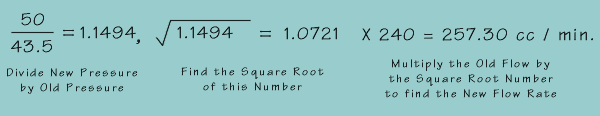Example 2:
The horsepower value of this injector would increase by the same rate
(X 1.0721), if all other engine management systems remain unchanged.

 Flow rate at old pressure 240 cc/Min divided by 10.5 = 22.86 Lb/Hr Flow rate at new pressure 257 cc/Min divided by 10.5 = 24.48 Lb/Hr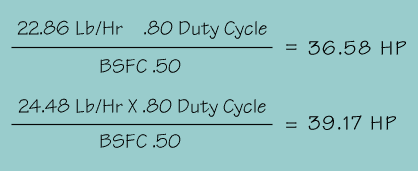So, the resultant change in pressure would yield the following:
240 cc/Min X 1.0721 = 257 cc/Min
22.86 Lb/Hr X 1.0721 = 24.51 Lb/Hr
36.58 Hp X 1.0721 = 39.22 Hp

###### Conversion Formulas

To convert cc / min to lbs. / hr. - Divide by 10.5
To convert lbs. / hr to gal. / hr. - Divide by 6
To convert cc / min to gal. / hr. - Multiply by .015873

#### Fuel Injector Worksheet

* See notes below on which BSFC value to use.

Warning: Duty cycle should not exceed 80% (0.80) for these calculations.

Lbs/Hr       CC/Min

* Brake specific should be .45 to .50 for naturally aspirated engines,
.55 TO .60 for supercharged engines, and .60 to .65 for turbocharged engines.